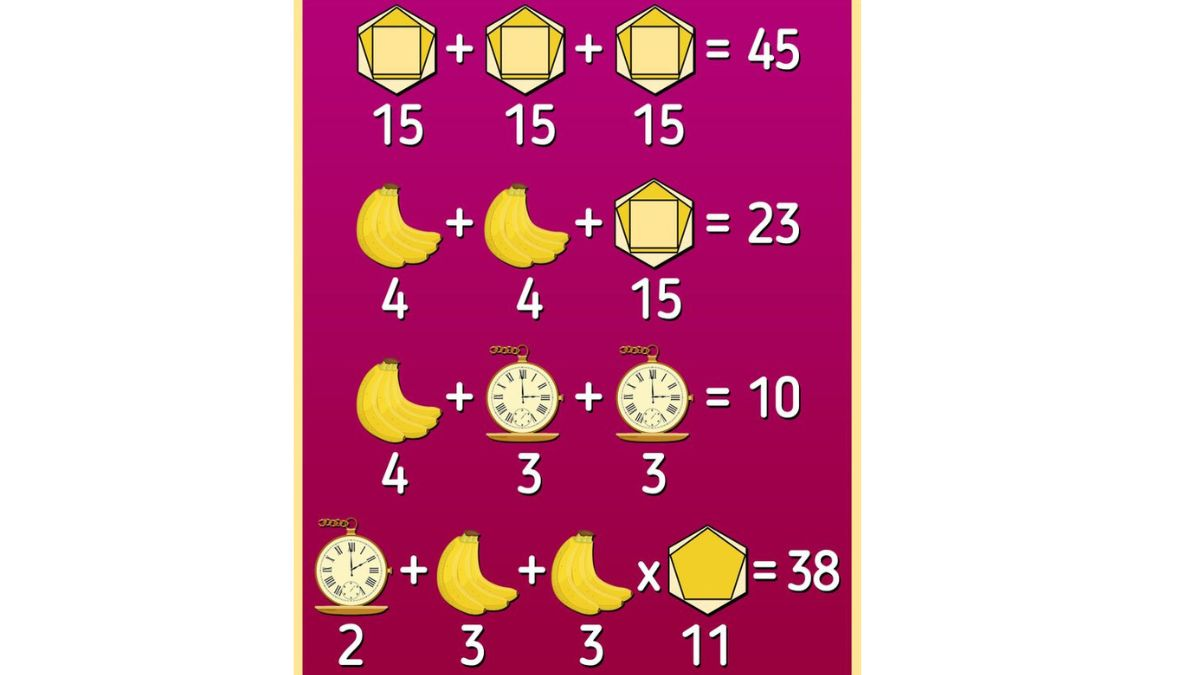# Brain Teaser: Can you solve this Elementary Level Mathematics Equation within 3 Minutes?

Enough of colors, patterns, and odd ones out, now it's time to solve some digits equations. Take this challenge to test your quantitative skills. Time Restrictions Applied.Can you solve this elementary level Math Puzzle?

No sun on Monday turns the Blues even stronger. But we can surely help you with it, just try your hand at this math puzzle. A Mathematical brain teaser is typically a puzzle that requires creativity, and great observational skills along with mathematical formulas. Also, this mathematical puzzle will strengthen the connection between brain cells, increase mental agility, and will help with short-term memory issues.
Source: Brightside.com

## Can you find the answer to this mathematical puzzle?

Brain Teasers require an unconventional way of thinking, however, sometimes it also involves an excellent quantitative approach. In contrast to the above image, you must use your observational skills and visual sharpness to solve this equation. Although the equation really simple, you just need to recall those easy formulas of elementary classes.

True to its name, Brain Teaser, it will be tricky, but still don’t look for the answer without attempting your luck twice.

Can you guess who is not pregnant in this picture?

## Look for the Math Puzzle answer here:

A math puzzle is essentially a formula and logic-based thinking brain teaser. As a result, with each trial, your ability to think and make decisions will get better. The brain game will also aid in problem-solving and strengthening your critical intuition.

The goal for you here is to find the value of the equation in respect of the given variables. Easy, right?

But I forgot to tell you, you just have 3 minutes to solve this brain teaser.

Tick…

Tock…

Tick…

The image shows three solved and one unsolved equation of elementary classes. And your task is to find a solution for the last with the given value of patterns and objects.

Let’s find the value of each equation

First Equation

Assume the value of each object as x

Hence,

X+X+X = 45

3x = 45

x= 45/3

x= 15

Second Equation

Let’s assume the value of bananas = Y

Y + Y + 15 = 23

2Y = 23- 15

Y = 8/2

Y = 4

Third Equation

Let’s assume the value of Pocket Watch = Z

4 + Z + Z = 10

4+ 2Z = 10

2Z = 10-4

Z= 6/2

Z= 3

Now, the final equation

Z + Y + Y x X =?

Keep all the estimated values in place

Value of Pocket Watch= 2 (Exactly, what it is ticking)

Value of Bananas= 3 (Count the number of bananas)

Value of Pattern= 11 ( 6 of hexagon and 5 of Pentagon)

2 + 3 + 3 x 11

Use BODMAS Rule…

Hence,

2+3+33 + 38

Are you still, struggling? Then please have a look at the picture below to know the accurate answer for this brain teaser.

Source: Brightside.com

I am sure, this was enough to shoo your Monday Blues. Also, keep a tab on these brain teasers to improve your cognitive, and problem-solving skills and memory sharpness.

Only people of detective skills can spot the father of infant.

Get the latest General Knowledge and Current Affairs from all over India and world for all competitive exams.
खेलें हर किस्म के रोमांच से भरपूर गेम्स सिर्फ़ जागरण प्ले पर